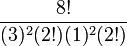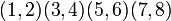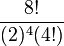# Element structure of symmetric group:S8

## Contents

View element structure of particular groups | View other specific information about symmetric group:S8

For convenience, we take the underlying set to be$\{ 1,2,3,4,5,6,7,8\}$.

## Conjugacy class structure

FACTS TO CHECK AGAINST FOR CONJUGACY CLASS SIZES AND STRUCTURE:
Divisibility facts: size of conjugacy class divides order of group | size of conjugacy class divides index of center | size of conjugacy class equals index of centralizer
Bounding facts: size of conjugacy class is bounded by order of derived subgroup
Counting facts: number of conjugacy classes equals number of irreducible representations | class equation of a group

### Interpretation as symmetric group

FACTS TO CHECK AGAINST SPECIFICALLY FOR SYMMETRIC GROUPS AND ALTERNATING GROUPS:
Conjugacy class parametrization: cycle type determines conjugacy class (in symmetric group)
Conjugacy class sizes: conjugacy class size formula in symmetric group
Other facts: even permutation (definition) -- the alternating group is the set of even permutations | splitting criterion for conjugacy classes in the alternating group (from symmetric group)| criterion for element of alternating group to be real

For a symmetric group, cycle type determines conjugacy class, so the conjugacy classes are parametrized by the set of unordered integer partitions of the number 8.

Partition Verbal description of cycle type Representative element Size of conjugacy class Formula for size Even or odd? If even, splits? If splits, real in alternating group? Element orders
1 + 1 + 1 + 1 + 1 + 1 + 1 + 1 all points fixed$()$ -- the identity element 1$\frac{8!}{(1)^8(8!)}$ even;no 1
2 + 1 + 1 + 1 + 1 + 1 + 1 transposition, six fixed points$(1,2)$ 28$\frac{8!}{(2)(1)^6(6!)}$, also$\binom{8}{2}$ odd 2
3 + 1 + 1 + 1 + 1 + 1 one 3-cycle, five fixed points$(1,2,3)$ 112$\frac{8!}{(3)(1)^5(5!)}$ even;no 3
4 + 1 + 1 + 1 + 1 one 4-cycle, four fixed points$(1,2,3,4)$ 420$\frac{8!}{(4)(1)^4(4!)}$ odd 4
2 + 2 + 1 + 1 + 1 + 1 two transpositions, four fixed points$(1,2)(3,4)$ 210$\frac{8!}{(2)^2(2!)(1)^4(4!)}$ even;no 2
5 + 1 + 1 + 1 one 5-cycle, three fixed points$(1,2,3,4,5)$ 1344$\frac{8!}{(5)(1)^3(3!)}$ even;no 5
3 + 2 + 1 + 1 + 1 one 3-cycle, one transposition, two fixed points$(1,2,3)(4,5)$ 1120$\frac{8!}{(3)(2)(1)^3(3!)}$ odd 6
6 + 1 + 1 one 6-cycle, two fixed points$(1,2,3,4,5,6)$ 3360$\frac{8!}{(6)(1)^2(2!}$ odd 6
4 + 2 + 1 + 1 one 4-cycle, one 2-cycle, two fixed points$(1,2,3,4)(5,6)$ 2520$\frac{8!}{(4)(2)(1)^2(2!)}$ even;no 4
2 + 2 + 2 + 1 + 1 three 2-cycles, two fixed points$(1,2)(3,4)(5,6)$ 420$\frac{8!}{(2)^3(3!)(1)^2(2!)}$ odd 2
3 + 3 + 1 + 1 two 3-cycles, two fixed points$(1,2,3)(4,5,6)$ 1120$\frac{8!}{(3)^2(2!)(1)^2(2!)}$ even;no 3
7 + 1 one 7-cycle, one fixed point$(1,2,3,4,5,6,7)$ 5760$\frac{8!}{(7)(1)}$ even;yes;no 7
3 + 2 + 2 + 1 one 3-cycle, two transpositions, one fixed point$(1,2,3)(4,5)(6,7)$ 1680$\frac{8!}{(3)(2)^2(2!)(1)}$ even;no 6
4 + 3 + 1 one 4-cycle, one 3-cycle, one fixed point$(1,2,3,4)(5,6,7)$ 3360$\frac{8!}{(4)(3)(1)}$ odd 12
5 + 2 + 1 one 5-cycle, one 2-cycle, one fixed point$(1,2,3,4,5)(6,7)$ 4032$\frac{8!}{(5)(2)(1)}$ odd 10
2 + 2 + 2 + 2 four 2-cycles$(1,2)(3,4)(5,6)(7,8)$ 105$\frac{8!}{(2)^4(4!)}$ even;no 2
4 + 2 + 2 one 4-cycle, two 2-cycles$(1,2,3,4)(5,6)(7,8)$ 1260$\frac{8!}{(4)(2)^2(2!)}$ odd 4
3 + 3 + 2 two 3-cycles, one 2-cycle$(1,2,3)(4,5,6)(7,8)$ 1120$\frac{8!}{(3)^2(2!)(2)}$ odd 6
6 + 2 one 6-cycle, one 2-cycle$(1,2,3,4,5,6)(7,8)$ 3360$\frac{8!}{(6)(2)}$ even;no 6
5 + 3 one 5-cycle, one 3-cycle$(1,2,3,4,5)(6,7,8)$ 2688$\frac{8!}{(5)(3)}$ even;yes;no 15
4 + 4 two 4-cycles$(1,2,3,4)(5,6,7,8)$ 1260$\frac{8!}{(4)^2(2!)}$ even;no 4
8 one 8-cycle$(1,2,3,4,5,6,7,8)$ 5040$\frac{8!}{8}$ odd 8